Impact Factor 2.638 | CiteScore 2.3
More on impact ›

# Frontiers in Physics## ORIGINAL RESEARCH article

Front. Phys., 04 September 2019 | https://doi.org/10.3389/fphy.2019.00122

# Drag Force for Asymmetrically Grafted Colloids in Polymer Solutions

• 1Department of Inhomogeneous Condensed Matter, Max-Planck-Institut für Intelligente Systeme, Stuttgart, Germany
• 2IV. Institut für Theoretische Physik, Universität Stuttgart, Stuttgart, Germany
• 3Institute of Physical Chemistry, Polish Academy of Sciences, Warsaw, Poland

We consider the situation in which a colloidal particle modifies locally the solvent leading to a spatially dependent viscosity. This situation is typical for colloidal particles in crowded environment, for example DNA-grafted particles in a polymer solution, or a hot particle which implies a temperature gradient to a viscous liquid. By means of suitable approximations we calculate the dependence of the friction force on the profile of the local viscosity. Our results show that in the case of axially symmetric viscosity profile the friction force is sensitive to the anisotropy of the viscous profile whereas it is not sensitive to for-ahead asymmetries. Our results are crucial for active microrheology measurements where tracer particles are pulled through complex fluids.

## 1. Introduction

Particles in the nanometer size range coated with polymers are of growing importance for rather diverse applications . In hybrid materials such as nanocomposites, the use of polymers grafted to nanoparticles is widely exploit to suppress aggregation of particles and to enhance their dispersion and mixing into solvent or matrix. The nanoparticles coated with DNA are used for building highly sensitive probes or drug carriers in biological systems [2, 3] and to assemble crystals and other structures of numerous morphologies .

In the absence of external driving, the transport of nanobjects in the fluid environment is dominated by diffusion, a process due to random molecular motion excited by thermal fluctuations . Diffusion of isolated spherical nanoparticle in the simple molecular liquids is well-described by the Fick's law, which says that the mean-square displacement changes linearly in time. The rate of this change, the translational diffusion coefficient Dt, is related to the macroscopic viscosity of the solvent ηm (as measured rheometer) via Stokes-Sutherland-Einstein (SSE) relation [6, 8]; Dt = kBTm where ζm is the hydrodynamic drag coefficient given by the Stokes equation ζm = 6πηmR. In this equation R is the hydrodynamic radius of diffusing particle, kB is the Boltzmann constant and T is the temperature.

However, various experiments  and simulation studies  show that diffusion of nano-sized particles in complex fluids is not accurately described by Fick's law and that the SSE relation is violated in certain regimes of parameters. For polymer solutions, these parameters involve the size of the particle and the polymer length scales [24, 25]. For example, if the particle size is comparable to or smaller than the characteristic length scale in a polymer solution, its diffusion is significantly faster than the one predicted based on the macroscopic viscosity [13, 17, 18]. This is because on such length scales the nanoparticle does not experience the homogeneous continuum medium with high viscosity, rather, the individual polymer chains or blobs and their fluctuations as well as entanglements influence its dynamics.

Grafting nanoparticles with macromolecules, such as polymers or DNA, complicates their interactions with the complex medium and, therefore, stronger deviations from the SSE relation are expected. These deviations can be tested in rheological experiments  by measuring the drag force. The experiments for a bare (non-grafted) colloid in DNA solutions demonstrated that by using optical tweezers it is possible to move a particle through a highly monodispersed polymer solution at a given velocity as well as to measure the drag force on the colloid with piconewton resolution at the same time .

Here we provide a theoretical prediction for a drag force based on the assumption that interactions between grafted particle and the complex medium result in an effective spatially dependent viscosity. A similar idea was used by Tuinier et al. , Fan et al. [28, 29], and Feng et al.  to calculate the hydrodynamic resistance force for a bare spherical particle in a non-adsorbing polymer solution, where polymer depletion results in a reduced polymer concentration near the particle surface. Using the concept of local viscosity , the polymer concentration profile was related to the viscosity profile near a spherical particle. Hydrodynamics was formulated by the modified Stokes equation with non-uniform spherically symmetric viscosity, which was solved by a regular perturbation approximation using the Green function method.

In the present paper, we extend this analysis to account for anisotropic viscosity profile around a spherical particle, which may result from anisotropically grafted nanoparticles in a non-adsorbing polymer solution (see Figure 1). Such anisotropically grafted nanoparticles, e.g., DNA-grafted Janus particles can be manufactured . They provide a basic structural element that can be used to produce useful nanoparticle clusters of different topologies through DNA-based self-assembly . The concept of anisotropic viscosity might also be applicable for describing the transport of protein through the nuclear pore complex, where the transporting protein encounters heterogeneous polymer brush or gel like environment .

FIGURE 1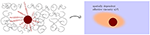Figure 1. Sketch of an anisotropic polymer concentration profile around a DNA-grafted colloid in a non-adsorbing polymer solution. The concentration profile of the free polymers depends on the grafting density and length of the DNA strings . The anisotropic concentration profile leads to a non-uniform viscosity variation. We want to stress that the system is in equilibrium and there is no netforce.

Because the concentration profile of free polymers in the solution depends on the length of grafted macromolecules and on the grafting density , an anisotropic grafting changes the depletion zone around the particle, which leads to anisotropic polymer concentration and thus to anisotropic effective viscosity. We assume that the particle is dragged slow enough such that the polymer solution can adiabatically follow the motion of the colloid. This means that the advective transport rate must be smaller than the diffusive transport rate of the polymers, which can be expressed via the Peclét number Pe = lpu/Dp ≪ 1, where lp is the characteristic length scale of the polymer, u the characteristic velocity of the solvent u, and Dp diffusivity of the polymers. Further, we assume that the characteristic length scale of the variation of polymer concentration is bigger than the length of an effective statistical chain element. This assures a sufficiently large number of chain elements in small volume element to apply the concept of local viscosity . Solving the modified Stokes equations with a spatial-dependent viscosity of a general form is not easy—even within a regular perturbation approximation. However, for the axisymmetric systems some simplifications occur. For axisymmetric Stokes equations with constant viscosity, translational and rotational motion are decoupled. For translational motion a scalar stream function, which transforms vectorial equations to the scalar ones is well-established. We demonstrate that these properties also hold for the modified Stokes equations with axisymmetric viscosity profile and provide a formalism to calculate the drag force experienced by a translating particle.

Our paper is structured as follows. In section 2, we present the extended Stokes equations with the spatially dependent viscosity and introduce the perturbation calculation scheme. In section 3 the drag force for the axisymmetric systems is calculated and analyzed for various grafting geometries. We conclude in section 5.

## 2. Model

### 2.1. Extended Stokes Equation

The Stokes equations are valid for small Reynolds numbers Re = ρul/η ≪ 1, where ρ is the density of the fluid, u is the characteristic velocity of the flow, and l is the characteristic length scale of the particle. This assures that viscous forces dominate the inertial forces. The stationary incompressible Stokes equations with spatially dependent viscosity η(r) are given by

where τ = −pI + 2η(r)Δ the stress tensor, p is a pressure and Δ = [v + (v)T]/2 is the strain rate. The superscript T denotes the transposed of a tensor, I is the identity matrix. Expanding the divergence in the momentum equation one obtains an additional term, which is proportional to the gradient of the viscosity

We consider a quiescent, unbounded fluid which is dragged by a particle with no-slip and vanishing far-field boundary conditions:

where Σp is the particle surface and rp is a point on the particle surface Σp. The particle is translating with velocity U and rotating with angular velocity Ω, which requires a force F and a torque T acting on the particle. The angular motion of the particle is described in a coordinate system fixed to the particle at rc.

The drag force on the particle is determined by the force acting on the particle surface Σp, which corresponds to the momentum flux through the surface

where n is the surface normal vector. In the following all quantities are represented in dimensionless units: vU, ΩU/a, $\tau \propto \stackrel{-}{\eta }U/a$ and $p\propto \stackrel{-}{\eta }U/a$, where a is the particle radius and $\stackrel{-}{\eta }$ is the bulk viscosity. Accordingly, the drag force and viscosity are also dimensionless.

### 2.2. Small Viscosity Variations

We consider an expansion of the system in small viscosity perturbations of order ${O}\left(ϵ\right)$

The fluid velocity at the surface of the particle is determined by the leading order velocity field v0. Thus, the higher order fluid velocity fields at the surface must vanish. The far-field condition of the quiescent fluid requires that both the pressure and the velocity fields have to vanish at infinity.

We expand the stationary Stokes equations in small viscosity variations of order ϵ. The leading order system ${O}\left({ϵ}^{0}\right)$ is given by

and the first order system ${O}\left({ϵ}^{1}\right)$

The leading order solutions fulfill common Stokes equations with constant viscosity.

## 3. Axisymmetric Systems

Axisymmetric systems possess at least one axis of rotational symmetry, which we choose to be parallel to ez. We use spherical coordinates {r, θ, ϕ} with the corresponding orthonormal basis $\left\{\stackrel{^}{r},{e}_{\theta },{e}_{\varphi }\right\}$ and the origin located at the center of the particle. Due to the rotational symmetry the system is independent of the azimuthal angle ϕ. Thus, the viscosity η(r, θ) is a function of radial distance r and the polar angle θ. Accordingly, the $\stackrel{^}{r}$- and eθ-components of the extended Stokes equations Equation (1) depend on the components vr and vθ of the fluid velocity, whereas the eϕ-component of Equation (1) depends only on vϕ. The velocity field of the fluid for translational motion of the particle Uez is determined by {vr, vθ} and for rotational motion Ωez is determined by vϕ. These properties are summarized in Figure 2.

FIGURE 2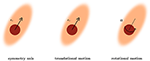Figure 2. Short summary of the decoupling of translational and rotational motion of the extended Stokes equations for an axisymmetric system. The velocity field of the fluid for translational motion of the particle Uez is determined by {vr, vθ} and for rotational motion Ωez is determined by vϕ.

For axisymmetric Stokes equations translational and rotational motion are decoupled. For translational motion a scalar stream function formalism is well-established to simplify the vectorial equation to a scalar one (see, e.g., Happel and Brenner ). This formalism can be extended to a non-uniform viscosity as long as it is axisymmetric (see Appendix A). In the following we focus on translational motion of a particle in an axisymmetric system which is characterized by the particle velocity U.

### 3.1. Leading Order Solution

The leading order axisymmetric momentum equation [see (A5) in the Appendix A] is the same as for the homogeneous system. So the stream function (see Equation A1) for the leading order system is 

The corresponding velocity field is given by

As expected, the leading order decay of the velocity is ∝ 1/r. The pressure is determined by integration

### 3.2. First Order Solution

For the first order velocity field we use Equations (B3) (see Appendix B), which correspond to the stream function ansatz (B2). In order to determine the first order pressure contribution p1(r = 1, θ) at the particle surface, we exploit the eθ-component of the stationary Stokes equation

Using the stream function relations for v0 (9) and v1 (B3) we integrate over θ to obtain the pressure at the surface r = 1

The integration constant c(r) does not contribute to the drag force. Here, superscript (i) defines the ith derivative.

### 3.3. Drag Force

The drag force on the particle is calculated by integrating the stress tensor over the particle surface (see Equation 4). First, we derive an expression for the forces fs(r, θ) at the particle surface r = 1

($\stackrel{^}{r}$ is a vector normal to the surface denoted in Equation 4 by n). Up to the first order, the surface force can be expressed as fs = fs0 + ϵfs1. Using solutions for zero and first order velocities and pressures derived above, we find

In the leading order, and f0seθ = 3/2 sin θ. It turns out that the orthogonal component ${f}_{s}·\stackrel{^}{r}$ of the surface force is determined by the pressure whereas the tangential component fs·eθ is determined by the shear force contribution.

The last step to calculate the drag force F is to integrate the surface forces over the whole surface of the particle $F={\int }_{{\Sigma }_{p}}\text{dS}{f}_{s}$. Due to the symmetry of the system the drag force is aligned parallel to the symmetry axis ez. The resolved part of the surface force fs in ez direction is obtained by using and ez = −eθ sin θ. Performing partial integration and applying the orthogonality of Gegenbauer functions (C2) as well as relation (C3) (see Appendix C) the drag force can be expressed as

The first line (14a) is the leading order drag force corresponding to a sphere and a homogeneous viscosity. The negative sign shows that the force is opposite to the particle moving direction. It turns out that in the leading order, the pressure contributes 1/3 and the shear force 2/3 of the total drag force. The last two lines (14b) and (14c), give the first order correction to the drag force. The contribution of the first order pressure at the surface is shown in line (14b) and the first order shear force contribution is shown in line (14c). Equation (13) implies that only the second Gegenbauer coefficient f2 is needed to calculate the first order drag force. A general solution for f2 can be provided by the Green function of the ODE (14) with n = 2, which has a form

For a sphere in an unbounded quiescent fluid with vanishing velocity at the sphere surface, this Green function has been calculated by Tuinier et al. :

The coefficient f2 is obtained via integration of the Green function and the second Gegenbauer mode of the inhomogeneity R2(r)

It satisfies the following boundary conditions: at the sphere surface ${f}_{2}\left(r=1\right)=0,{f}_{2}^{\left(1\right)}\left(r=1\right)=0,$ and in far-field $\underset{r\to \infty }{lim}:{f}_{2}\left(r\right)/{r}^{2}=0,{f}_{2}^{\left(1\right)}\left(r\right)/r=0$. All integrands in (17) are smooth functions, therefore, even if for a given r-dependence of the viscosity an analytical solution does not exist, the integrals can be easily calculated using the standard quadrature method. For the first order drag force, also the derivatives f(2)(r = 1) and f(3)(r = 1) at the surface of the sphere are needed. Considering the Leibnitz rule and the continuity of the given Green function up to the second derivative, the derivatives of f2 at the surface are

#### 3.3.1. Multipol Representation

In order to proceed, we represent the first order viscosity variation in Equation (5a) as a multipole expansion with r-dependent coefficients

In the above, the symbol “:” denotes the double dot product. The projections of the viscosity variation are: the monopole , the dipole and the quadrupole . The quadrupole matrix Q is traceless and symmetric by definition. It follows that the second Gegenbauer coefficient R2(r) of the inhomogeneity h1(r, θ) in Equation (B4) depends on the monopole and quadrupole contributions to the axisymmetric viscosity but does not depend on the dipole contribution. Thus, for the total drag force F one has

In the above

$𝒢(r′)≡(1r′6-3r′4), ∫1∞dr′𝒢(r′)=-45.$

This result includes the solution of Tuinier et al.  for isotropic viscosity variations η1(r).

## 4. Analysis

We start the analysis of our result for the drag force with the general case of viscosity variations that decay beyond a certain length scale. The decay of viscosity variations is expected because the overall polymer concentration profile approaches its bulk value sufficiently far away from the grafted particle. Specifically, we assume that each multipole mode n of η1 decays beyond the (possibly different) length scale dn. Since merely the first three multipoles are relevant, we have

In order to grasp the basics of the role of the viscosity profile on the drag force on the colloid, we follow Tuinier and Taniguchi  and consider the decay profile of the following functional form:

with the properties Zn(r = 1; dn) = 1, ${Z}_{n}^{\left(1\right)}\left(r=1;{d}_{n}\right)=0$, and $\underset{r\to \infty }{lim}{Z}_{n}\left(r;{d}_{n}\right)=0$. An example of the decay profile is shown in Figure 3 for different values of d.

FIGURE 3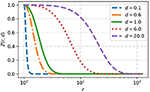Figure 3. The decay profile Z(r; d) next to the particle surface r = 1 is plotted for different d ∈ {0.1, 0.6, 1, 8, 20}. d < 1 corresponds to a fast decay compared to the particle size and d > 1 corresponds to a slow decay.

The drag force can be represented in terms of an isotropic viscosity contribution ϵm αi(d) and the anisotropic viscosity contributions ϵQ33 αa(d) as follows:

with

In Figure 4 we plot the forces αi and αa as function of the decay length d ∈ [10−3, 103]. As expected, for variations very quickly decaying with the distance from the sphere, i.e., for (d ≪ 1), both functions vanish and the drag force is similar to a particle in a homogeneous solution. Thus, in this limit the viscosity variation is negligible. On the other hand, in the limit of very slow decays (d ≫ 1)

the solution correspond to the case of viscosity variations that depend only on the angle θ.

FIGURE 4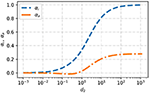Figure 4. Dimensionless drag force due to a solely monopole contribution, αi (see Equation 27), and solely due to quadrupole contribution, αa (see Equation 29), as a function of the dimensionless decay length, d2.

In between there is a transition region from short length scales to long length scales. The isotropic viscosity variation is the main contribution to the drag force. The contribution from the anisotropic viscosity variation is weaker. At d ≈ 1 the anisotropic contribution becomes negligibly small because αa shows a zero crossing in that region. Thus, up to the first order the drag force is independent of anisotropic viscosity variations that decay on the length scale of the particle. In order to get a better understanding of the zero crossing we look at the specific quadrupole like viscosity variation Q = [(−1, 0, 0), (0, −1, 0), (0, 0, 2)]/2, which is shown in Figure 5 for d2 ∈ {0.3, 7}. This specific viscosity variation does not change the net viscosity. The viscosity in front and at the back of the particle is increased whereas at the waist it is decreased. The corresponding drag force is increased for d2 > 1 and decreased for d2 < 1. In a more general context this indicates that higher viscosity at the back and front of a particle leads to larger drag force if the viscosity variation decays on a longer length scale compared to the length scale of the particle and to a weaker drag force if the variation decays on a shorter length scale compared to the length scale of the particle.

FIGURE 5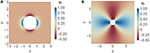Figure 5. Axisymmetric, quadrupolar viscosity variation Q = [(−1, 0, 0), (0, −1, 0), (0, 0, 2)]/2 around a spherical particle with length scale decay (A) d2 = 0.3 and (B) d2 = 7.

### 4.1. Analysis of the Velocity Mode vd

Our formalism allows us to analyze how the velocity field adapts to modulations in the local viscosity. In the following we focus on the dependence of the local velocity field on the local viscosity for anisotropic viscosity profiles (isotropic profiles have been analyzed in Fan et al. ). In this case only the quadrupolar component of the viscosity profile (with magnitude given by Q33 = l2, where l2 is the coefficient of the projection of η1 on the second Legendre mode1) affects the translational friction, thus we study the velocity field variations which are related to those viscosity variations and call them vd ∝ ϵl2.

In Figure 7 we show the velocity field variation vd (calculated using Equation 16) for different decay length of the quadrupolar contribution d2 ∈ {0.1, 1, 8, 20} (in units of ϵl2). For each decay d2 two figures are shown. The semi-logarithmic plot gives information about the long ranged velocity field vdz along the lines θ = 0 and θ = π/2 whereas the vector-density-plot indicates the absolute value of the velocity component |vd| and the arrows give additional information about the direction of the velocity variation. For comparison the leading order velocity field is shown in Figure 6.

FIGURE 6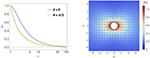Figure 6. Velocity profiles. The leading order velocity field is shown in two plots. The first one is a semi-logarithmic plot which gives information about the long ranged velocity field v0 decay parallel to the symmetry axis. Therefore we calculated v0 along the lines θ = 0 and θ = π/2. The second one is a vector-density-plot which indicates the absolute value of |v0| and the arrows give additional information about the direction of the velocity field. The particle is denoted by the white sphere in the middle of the plot and it is moving to the right parallel to ez.

In the semi-logarithmic plot we see the algebraic decay of the velocity field and at the surface r = 1 the dimensionless velocity of the particle v ∝ 1. Thus, a positive sign in the semi-logarithmic plot as well as arrows with positive z-component corresponds to an increase of the velocity field. On the other hand, a negative sign in the semi-logarithmic plot as well as arrows with negative z-component corresponds to a decrease of the velocity field. For short ranged viscosity variations d2 ≲ 1 the semi-logarithmic plot is negative as shown in the top right panel of Figure 7. Thus, the velocity variation decreases the velocity field. For viscosity variations of the particle length scale d2 ≈ 1 (middle left panel of Figure 7) the semi-logarithmic plot is mainly positive except close to the particle surface. Hence, the velocity variation increases the velocity field. This property is maintained in the far-field for long ranged viscosity variations. Finally, in the bottom right panel of Figure 7 the vector-density-plot shows two velocity regions which are separated by a crossover area (blue area) with very small velocity variations. The first region is close to the particle surface. Here the fluid velocity is reduced compared to the homogeneous case. The second region is outside the crossover area and increases the velocity field. With increasing length scale of the viscosity variation d the layers get stretched.

FIGURE 7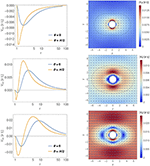Figure 7. Velocity profiles. The velocity field variation vd is shown for different decays of the viscosity variation d2 ∈ {0.1, 1, 8} top to bottom, respectively. Each velocity field is shown in two plots. The first one is a semi-logarithmic plot which gives information about the long ranged velocity field vdz decay parallel to the symmetry axis. Therefore we calculated vdz along the lines θ = 0 and θ = π/2. The second one is a vector-density-plot which indicates the absolute value of |vd| and the arrows give additional information about the direction of the velocity variation. The particle is denoted by the white sphere in the middle of the plot and it is moving to the right parallel to ez.

### 4.2. Janus and Quadrupole Particles

We consider a spherical particle that is anisotropically grafted with polymer chains in a polymer suspension. The grafted polymers induce an anisotropic depletion zone of suspended polymers whose width is controlled by the mutual interaction between the grafted and suspended polymers. In the following we assume that the motion of the particle occurs on time scales τparticle that are much larger than the relaxation time of the polymers (τparticle ≫ τpolymer), i.e., the Peclét number Pe = lpu/Dp ≪ 1.

Under these conditions the semi empirical Martin Equation  can be used to relate the local polymer concentration to a local viscosity:

where ρ is the dimensionless, normalized total polymer (i.e., grafted plus suspended polymers) concentration profile, ηs is the viscosity of the solvent, kH is the Huggins coefficient, which is specific for a given polymer-solvent combination. The intrinsic viscosity [η] is approximately the inverse of the polymer overlap concentration $1/{c}_{b}^{*}$ in the bulk, which corresponds to the hydrodynamic volume of a polymer chain in solution per unit mass. We expand the Martin equation for small variations of the polymer concentration profile cbρ(r), which is in the dilute regime ϵ = cb[η] ≪ 1

We identify η1 = ρ.

Now, we discuss the following (physically realizable) grafting geometries :

isotropic: bare particle – index ′i′;

dipolar grafting: a Janus like particle – index ′j′;

quadrupolar grafting two cases

– dominant length scale at back and front – index ′q′;

– dominant length scale at the waist – index $\prime {q}_{2}^{\prime }$;

which are defined by the following concentration profiles

The lowest monopoles of the viscosity corresponding to these concentration profiles are:

We assume that near a surface of the bare particle polymers are depleted, which corresponds to the polymer concentration profile 

The polymer concentration profile ρj next to a Janus like colloidal particle is shown in Figure 8A. The polymer concentration profile for the two cases ρq, ρq2 of quadrupolar grafting are shown in Figures 8A,C.

FIGURE 8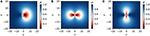Figure 8. Concentration profile ρ (see Equation 32) next to a dipolar grafted (Janus-like) colloidal particle (A), next to a quadrupolar back-front grafted colloidal particle (B), and a quadrupolar waist grafted particle (C). The length scale of the depletion zone at one hemisphere of the dipolar grafted particle and at the waist (back front) of the quadrupolar grafted particle is fixed at (d1 = 1) whereas at the other parts of the particle it is fixed at d = 10.

We calculate the drag force for different length scales d ∈ [0.01, 50] of the depletion zone at the grafted sides and compare it to the isotropic case. The length scale of the depletion zone at one hemisphere of the dipolar grafted particle, the waist of the quadrupolar back-front grafted particle ′q′ as well as the back and front of the waist grafted particle ($\prime {q}_{2}^{\prime }$) are fixed at d1 = 1 or at d1 = 0.01.

The results for the first order correction to the drag force Fz,1 = (Fz − 1)/(−6π) (in units of ϵ) are shown in Figure 9 for two cases: (1) d1 = 1 and (2) d1 = 0.01. One can see that for all cases Fz,1 is positive, i.e., the total drag force always increase, and that the first order correction decays monotonically upon increasing the decay length d. Because the dipolar part of the axisymmetric viscosity variations does not contribute to the first order correction to the drag force, for the Janus like grafting Fz,1 is determined by the monopol part (36). For the quadrupolar grafting both monopol and quadrupole viscosity variations contribute to Fz,1. By the choice of the polymer concentration profiles, at d1 = d the drag force Fz,1 for all cases of grafted particles equals the one for the bare particle. If d1 < d, i.e., when the grafting induce a larger depletion zone, the first order correction to the drag force is smaller than that for a bare particle. If d1 or d are < 1, Fz,1 is almost the same for all three cases of grafting. For d > d1, the back-front grafting leads to a weaker Fz,1 than the waist grafting.

FIGURE 9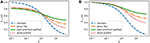Figure 9. The first order correction to the drag force Fz,1 = (Fz−1)/(−6π) (see Equation 25) for three cases of the grafting geometry as defined by Equations (32–34) compared to the case of isotropic (bare) particle Equation (31). Polymer concentration profiles corresponding to the drag force for the Janus and back-front quadrupolar grafting in (A) are shown, respectively, in panels (A,B) in Figure 8.

## 5. Conclusions

We have characterized the friction force of colloidal particles dragged across a fluid that show an inhomogeneous and anisotropic viscosity profile. We have derived closed formulas for both the drag force as well as for the velocity profile. In order to rationalize our results we have expressed the local viscosity profile in terms of its multipole expansion. As expected, we found that the drag force is sensitive to the amplitude of the monopole, i.e., the drag force increases upon increasing the average viscosity. Interestingly, higher order multipoles have quite different roles. In fact, our results show that the drag force is insensitive to the amplitude of the dipole, i.e., for a fore-and-aft asymmetric particle pulling it back and forwards leads to the same drag force. In contrast, the drag force is sensitive to the amplitude of the quadrupole. In particular, we found that a proper choice of the quadrupole orientation (i.e., with higher viscosity on the waist and lower on the axis of motion) with a long decay, d ≫ 1 leads to a net reduction of the drag force. We emphasize that such a reduction occurs with “fixed” average viscosity, i.e., at fixed monopole contribution. Hence this is a genuine effect of the anisotropic viscosity distribution and cannot be reduced to a simple reduction of the average drag. Moreover, the sign of this contribution can be switched by changing either the sign of the quadrupole, i.e., moving the higher viscosity from the waist of the particle to the axis of motion, or by reducing the decay length, d ≪ 1.

We have analyzed the cases of physically plausible grafting shown in Figure 7 in more detail. Interestingly, we found that the net drag force can be controlled by tuning the relative magnitude of the monopole and quadrupole contribution to the density of the grafted polymers (see Figure 8). In particular, our results show that for the Janus grafting the anisotropic grafting can both reduce and enhance the drag force as compared to the isotropic case. In contrast, for the case of back-front grafting the net effect of the anisotropic contribution is to enhance the drag force as compared to the isotropic case.

Concerning the velocity profile, we have shown that the inhomogeneous and anisotropic viscosity profile induces quite involved modulations in the velocity field. Interestingly, the modulations of the velocity profile can oppose t the main flow. Due to our perturbation approach, the magnitude of these modulations are tiny. In this perspective, our results are pinpointing the relevance of the modulations in the viscosity that, possibly, may persist also for stronger variations of the viscosity. We have truncated our expansion at first order since, typically, this is also the leading order when the perturbation parameter is small. Indeed this is the case for monopole and quadrupole contributions to the density of grafted polymers. Interestingly, dipole contributions to the density distribution, at linear order, do not affect the effective friction. Therefore, for the dipolar contribution a higher order expansion is required. Since this quadratic contribution is relevant only when the monopole and quadrupole are vanishing small, we have decided to disregard this contribution in the present manuscript and to focus on the leading contributions, namely monopole and quadrupole. We plan to investigate higher order contribution (and hence also dipole ones) in forthcoming works. Results of Fan et al.  suggest that they may be relevant for variations at short length scales.

## Data Availability

The datasets generated for this study are available on request to the corresponding author.

## Author Contributions

MW, PM, and AM contributed conception and design of the study. MW wrote the first draft. AM rewrote several sections. All authors contributed to manuscript revision.

## Conflict of Interest Statement

The authors declare that the research was conducted in the absence of any commercial or financial relationships that could be construed as a potential conflict of interest.

## Acknowledgments

The work of AM was partially supported by Polish National Science Center (Harmonia Grant No. 2015/18/M/ST3/0403).

## Supplementary Material

The Supplementary Material for this article can be found online at: https://www.frontiersin.org/articles/10.3389/fphy.2019.00122/full#supplementary-material

## Footnotes

1. ^A function f defined on the interval [−1, 1] can be represented in Legendre's polynomials $f\left(x\right)=\sum _{n\ge 0}{l}_{n}{P}_{n}\left(x\right)$. The coefficients ln are the projections onto the corresponding Legendre's mode $\frac{2n+1}{2}{〈f\mid {P}_{n}〉}_{P}={l}_{n}$.

## References

1. Mezzenga R, Ruokolainen J. Nanocomposites: nanoparticles in the right place. Nat Mater. (2009) 8:926–8. doi: 10.1038/nmat2576

2. Rosi NL, Mirkin CA. Nanostructures in biodiagnostics. Chem Rev. (2009) 105:1547. doi: 10.1021/cr030067f

3. Tiwari PM, Vig K, Dennis VA, Singh SR. Functionalized gold nanoparticles and their biomedical applications. Nanomaterials. (2011) 1:31–62. doi: 10.3390/nano1010031

4. Nykypanchuk D, Maye MM, van der Lelie D, Gang O. DNA-guided crystallization of colloidal nanoparticles. Nature. (2008) 451:549–52. doi: 10.1038/nature06560

5. Brown R. In: Bennett JJ, Hardwicke R, editors. The Miscellaneous Botanical Works of Robert Brown. Vol. 1. London (1866). p. 463–86.

Google Scholar

6. Einstein A. Investigations on the Theory of the Brownian Movement. New York, NY: Dover (1956).

Google Scholar

7. Smoluchowski M. Zur kinetischen theorie der brownschen molekularbewegung und der suspensionen. Ann Phys. (1906) 21:756–80.

Google Scholar

8. Sutherland W. A dynamical theory of diffusion for non-electrolytes and the molecular mass of albumin. Philos Mag. (1905) 9:781–85.

Google Scholar

9. Odijk T. Depletion theory of protein transport in semi-dilute polymer solutions. Biophys J. (2000).79:2314–21. doi: 10.1016/S0006-3495(00)76477-0

10. Kalwarczyk T, Sozanski K, Ochab-Marcinek A, Szymanski J, Tabaka M, Hou S, et al. Motion of nanoprobes in complex liquids within the framework of the length-scale dependent viscosity model. Adv Colloid Interface Sci. (2015) 223:55–63. doi: 10.1016/j.cis.2015.06.007

11. Wong IY, Gardel ML, Reichman DR, Weeks ER, Valentine MT, Bausch AR, et al. Anomalous diffusion probes microstructure dynamics of entangled F-actin networks. Phys Rev Lett. (2004) 92:178101. doi: 10.1103/PhysRevLett.92.178101

12. Banks DS, Fradin C. Anomalous diffusion of proteins due to molecular crowding. Biophys J. (2005) 89:2960–71. doi: 10.1529/biophysj.104.051078

13. Tuteja A, Mackay ME, Narayan S, Asokan S, Wong MS. Hydroxylated quantum dots as luminescent probes for in situ hybridization. Nano Lett. (2006) 7:1276–81. doi: 10.1021/nl070192x

CrossRef Full Text

14. Saxton MJ. A biological interpretation of transient anomalous. Biophys J. (2007) 92:1178–91. doi: 10.1529/biophysj.106.092619

15. Grabowski CA, Adhikary B, Mukhopadhyay A. Dynamics of gold nanoparticles in a polymer melt. Appl Phys Lett. (2009) 94:021903. doi: 10.1063/1.3070533

16. Ziȩbacz N, Wieczorek SA, Kalwarczyk T, Fiałkowski M, Hołyst R. Crossover regime for the diffusion of nanoparticles in polyethylene glycol solutions: influence of the depletion layer. Soft Matter. (2011) 7:7181–6. doi: 10.1039/C0SM01357A

17. Kohli I, Mukhopadhyay A. Diffusion of nanoparticles in semidilute polymer solutions: effect of different length scales. Macromolecules. (2012) 45:6143–9. doi: 10.1021/ma301237r

18. Guo H, Bourret G, Lennox RB, Sutton M, Harden JL, Leheny RL. Entanglement-controlled subdiffusion of nanoparticles within concentrated polymer solutions. Phys Rev Lett. (2012) 109:055901. doi: 10.1103/PhysRevLett.109.055901

19. Chapman CD, Lee K, Henze D, Smith DE, Robertson-Anderson RM. Onset of non-continuum effects in microrheology of entangled polymer solutions. Macromolecules. (2014) 47:1181–6. doi: 10.1021/ma401615m

20. Grabowski CA, Mukhopadhyay A. Size effect of nanoparticle diffusion in a polymer melt. Macromolecules. (2014) 47:7238–42. doi: 10.1021/ma501670u

21. Ganesa V, Pryamitsyn V, Surve M, Narayanan B. Noncontinuum effects in nanoparticle dynamics in polymers. J Chem Phys. (2006) 124:221102. doi: 10.1063/1.2209241

22. Liu J, Cao D, Zhang L. Molecular dynamics study on nanoparticle diffusion in polymer melts: a test of the stokes Einstein law. J Phys Chem C. (2008) 112:6653–661. doi: 10.1021/jp800474t

23. Kalathi JT, Yamamoto U, Grest GS, Schweizer KS, Kumar SK. Nanoparticle diffusion in polymer nanocomposites. Phys Rev Lett. (2014) 112:108301. doi: 10.1103/PhysRevLett.112.108301

24. de Gennes PG. Scaling Concepts in Polymer Physics. Ithaca, NY: Cornel University Press (1979).

Google Scholar

25. Rubinstein M, Colby RH. Polymer Physics. New York, NY: Oxford University Press (2003).

Google Scholar

26. Gutsche C, Elmahdy MM, Kegler K, Semenov I, Stangner T, Otto O, et al. Micro-rheology on (polymer-grafted) colloids using optical tweezers. J Phys. (2011) 23:184114. doi: 10.1088/0953-8984/23/18/184114

27. Tuinier R, Dhont JKG, Fan TH. How depletion affects sphere motion through solutions containing macromolecules. Europhys Lett. (2006) 75:929–35. doi: 10.1209/epl/i2006-10200-0

28. Fan TH, Xie B, Tuinier R. Asymptotic analysis of tracer diffusivity in nonadsorbing polymer solutions. Phys Rev E. (2007) 76:051405. doi: 10.1103/PhysRevE.76.051405

29. Fan TH, Dhont JKG, Tuinier R. Motion of a sphere through a polymer solution. Phys Rev E. (2007) 75:011803. doi: 10.1103/PhysRevE.75.011803

30. Feng X, Chen A, Wang J, Zhao N, Hou Z. Understanding protein diffusion in polymer solutions: a hydration with depletion model. J Phys Chem B. (2016) 120:10114–23. doi: 10.1021/acs.jpcb.6b06248

31. Tuinier R, Taniguchi T. Polymer depletion-induced slip near an interface. J Phys. (2005) 17:L9–14. doi: 10.1088/0953-8984/17/2/l01

32. Wijmans CM, Zhulina EB, Fleer GJ. Effect of free polymer on the structure of a polymer brush and interaction between two polymer brushes. Macromolecules. (1994) 27:3238–48. doi: 10.1021/ma00090a017

33. Maye M, Nykypanchuk D, Cuisinier M, van der Lelie D, Gang O. Stepwise surface encoding for high-throughput assembly of nanoclusters. Nat Mater. (2009) 8:388–91. doi: 10.1038/nmat2421

34. Vivek MP, Steven DH. Nanoparticle assembly: DNA provides control. Nat Mater. (2009) 8:346–6. doi: 10.1038/nmat2436

35. Lattuada M, Hatton TA. Synthesis, properties and applications of Janus nanoparticles. Nano Today. (2011) 6:286–308. doi: 10.1016/j.nantod.2011.04.008

36. Chakrabarti R, Debnath A, Sebastian KL. Diffusion in an elastic medium: a model for macromolecule transport across the nuclear pore complex. Phys A. (2014) 404:65–78. doi: 10.1016/j.physa.2014.02.059

37. Mair A, Tung C, Cacciuto A, Coluzza I. Translocation of a globular polymer through a hairy pore. J Mol Liquids. (2018) 265:603–10. doi: 10.1016/j.molliq.2018.06.009

38. Chakrabarti R, Kesselheim S, Košovan P, Holm C. Tracer diffusion in a crowded cylindrical channel. Phys Rev E. (2013) 87: 062709. doi: 10.1103/PhysRevE.87.062709

39. Happel J, Brenner H. Low Reynolds Number Hydrodynamics. The Hague: Martinus Nijhoff (1983).

Google Scholar

40. Weissberg SG, Simha R, Rothman S. Viscosities of very dilute polymer solutions. J Res Natl Bur Stand. (1951) 47:2257.

Google Scholar

41. Fleer GJ, Skvortsov AM, Tuinier R. Mean-field equation for the depletion thickness. Macromolecules. (2003) 36:7857–72. doi: 10.1021/ma0345145

Keywords: crowded environments, polymer solution, drag force, anisotropic viscosity, transport phenomena and fluid mechanics, functionalized colloids

Citation: Werner M, Margaretti P and Maciołek A (2019) Drag Force for Asymmetrically Grafted Colloids in Polymer Solutions. Front. Phys. 7:122. doi: 10.3389/fphy.2019.00122

Received: 25 June 2019; Accepted: 14 August 2019;
Published: 04 September 2019.

Edited by:

Ralf Metzler, University of Potsdam, Germany

Reviewed by:

Rajarshi Chakrabarti, Indian Institute of Technology Bombay, India
Enzo Orlandini, University of Padova, Italy

Copyright © 2019 Werner, Margaretti and Maciołek. This is an open-access article distributed under the terms of the Creative Commons Attribution License (CC BY). The use, distribution or reproduction in other forums is permitted, provided the original author(s) and the copyright owner(s) are credited and that the original publication in this journal is cited, in accordance with accepted academic practice. No use, distribution or reproduction is permitted which does not comply with these terms.

*Correspondence: Anna Maciołek, maciolek@is.mpg.de